# NCERT Solutions for Class 3 Maths Chapter 12

## NCERT Solutions for Class 3 Maths Chapter 12 - Can We Share

Vedantu offers NCERT Solutions for Class 3 Maths Chapter 12 – Can We Share, designed by experienced teachers and subject experts as per the latest syllabus of CBSE Class 3. The NCERT Solutions for Class 3 Maths Chapter 12 PDF covers solutions for all the exercise questions given for this chapter in the NCERT textbook. Students can download these ‘NCERT Solutions Class 3 Maths Chapter 12- Can We Share?’ for free from Vedantu and go through them to secure good marks in the exam.

Do you need help with your Homework? Are you preparing for Exams?
Study without Internet (Offline)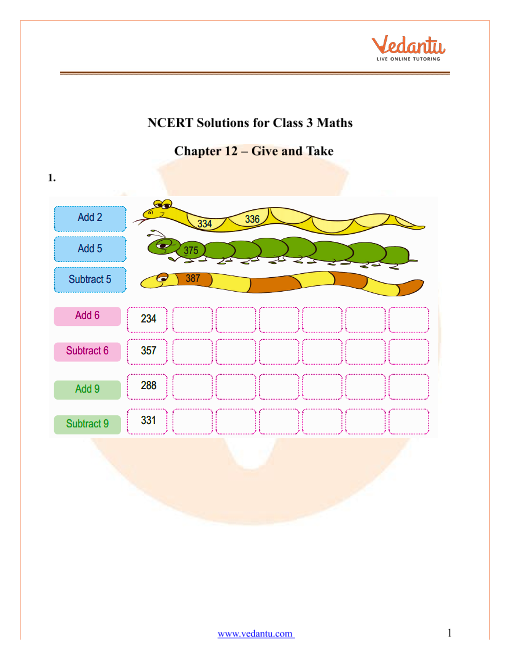## Access NCERT Solutions for Class 3 Mathematics Chapter 12 - Can We Share

1.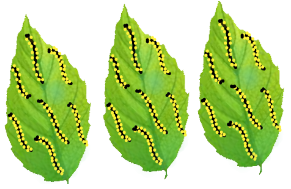There are ________ caterpillars. They are in __________ groups. There are _________ caterpillars in each group.

Ans:

There are $$21$$ caterpillars. There are $$3$$ groups. There are $$7$$ caterpillars in each group.

2.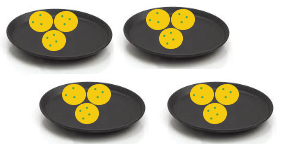There are __________ laddoos. They are in ________ groups. There are _________ laddoos in each group.

Ans:

There are $$12$$ laddoos. They are in $$4$$ groups. There are $$3$$ laddoos in each group.

3. Draw $$18$$ stars. Put them into $$2$$ equal groupThere are _________ stars in each group.

Ans: The total number of stars are $$18$$. To group it equally, divide $$18$$ by $$2$$. On dividing $$18$$ by $$2$$ we get $$9$$.There are $$9$$ stars in each group.

4. Draw $$18$$ beads. Put them into 3 equal groups.There are _________ beads in each group.

Ans: On dividing the $$18$$ by $$3$$, we get $$6$$There are $$6$$ beads in each group.

5. Now draw the jalebis on the plates below, so that each plate has the same number of jalebis.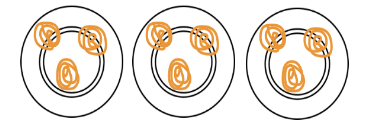How many jalebis are there altogether? _________ How many jalebis are there in each plate? _________ Discuss in the class how you found the answer.

Ans: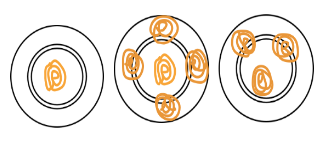There are $$9$$ jalebis all together. We have to distribute equally on  $$3$$ plates So we get $$3$$ jalebis in each plate. Given the maximum number of jalebis in a plate is $$5$$. The Jalebi in second plate are more when compared to first and third plate. There are $$5$$ jalebis in second plate  so we need to remove $$2$$  and transfer it in to first  plate  to make it as $$3$$ jalebis and keep jalebis as it is in third plate because it’s already have $$3$$ jalebis.

6. If there are $$60$$ bananas and two monkeys, how many will each monkey get? _________ bananas. What if there are $$600$$ bananas and two monkeys?

Ans: There are total $$60$$ bananas and two monkeys. If the monkeys divide the bananas equally then each monkey will get, on dividing $$60 \div 2 = 30$$.

Thus, each monkey will get $$30$$ bananas.

Suppose if there are $$600$$ bananas and it is to be divided among two monkeys then, the number of bananas each monkey will get, on dividing $$600$$ by $$2$$

$$600 \div 2 = 300$$

Thus, each monkey will get $$300$$ bananas.

7. Five friends found $$10$$ five-rupee coins on the ground.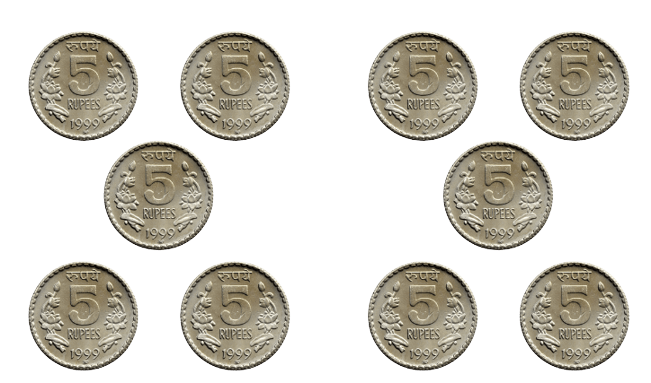They shared them equally. Each friend got ten rupees. $$50 \div 5 = 10$$ If there are $$16$$ ten-rupee notes and four friends to share, then $$16 \div 4 = 4$$ ________ and $$4 \times 10 = 40$$ so each friend gets ________ rupees.

Five friends found Rs $$100$$. If they share it equally, how much will each get? ________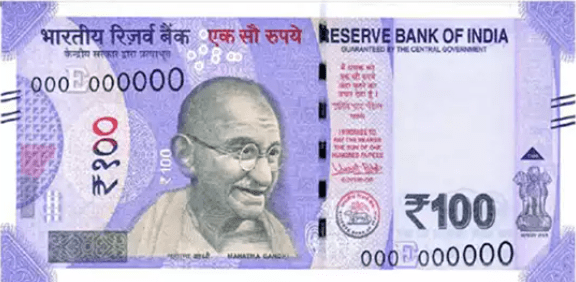Ans:

$$16 \div 4 = 4$$

$$4 \times 10 = 40$$

So, each friend gets $$40$$ rupees. $$100 \div 5 = 20$$ So, each friend will get $$20$$ rupees.

8. Hari prashad has 30 meters of rope. He distributes it equally among his three children. Each child gets ___________ meters of rope. If there is 36 meters of rope, how much of rope will each child get? ___________ And if there is 60 meters of rope, how much will each child get? ___________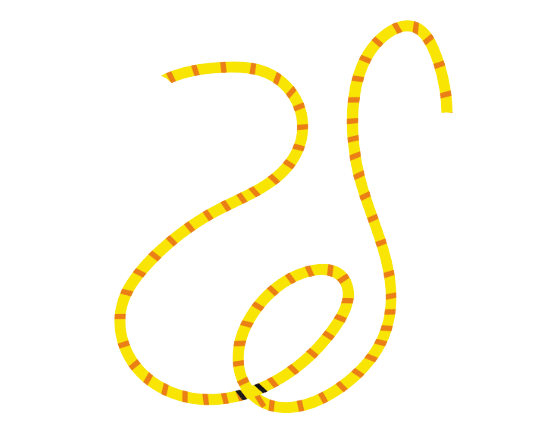Ans:

• Given, Hari prashad has length of the rope $$= 30$$meters

• Number of children getting the rope $$= 3$$

• Length of the rope each child gets $$= 30 \div 3 = 10$$ meters.

• Therefore, each child will get $$10$$ meters of rope.

• If, Hari prashad has length of the rope $$= 36$$meters

• Number of children getting the rope $$= 3$$

• Length of the rope each child gets $$= 36 \div 3 = 12$$ meters.

• Therefore, if there is $$36$$ meters of rope, then each child will get $$12$$ meters of rope.

• If, Hari prashad has length of the rope $$= 60$$meters

• Number of children getting the rope $$= 3$$

• Length of the rope each child gets $$= 60 \div 3 = 20$$ meters.

• Therefore, if there is $$60$$ meters of rope, then each child will get $$20$$ meters of rope.

9. Minku puts her 15 laddoos equally into 5 boxes.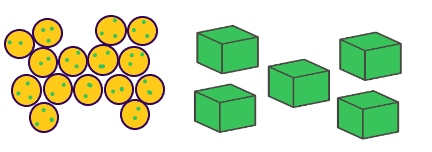i) How many ladoos will there be in each box? There will be __________ laddoos in each box. $$15 \div 5 =$$_________

ii) If she uses only 3 boxes, how many laddoos will there be in each box? There will be __________ laddoos in each box. ________ $$\div 3 =$$________

Ans:

i) Given, there are $$15$$ laddoos and $$5$$ boxes are to be filled with these laddoos. So, number of laddoos in each box $$= 15 \div 5 = 3$$

Hence, there will be $$3$$ laddoos in each box.

ii) If she uses only $$3$$ boxes.

Then, number of laddoos in each box $$= 15 \div 3 = 5$$

Hence, there will be $$5$$ laddoos in each box.

10. Share 25 bananas among 5 monkeys. How many bananas for each monkey?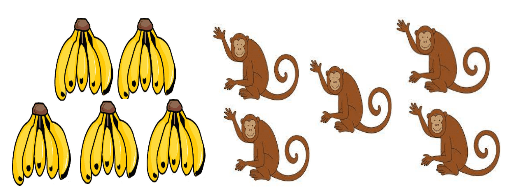______ $$\div 5 =$$ ______ Each monkey has _________ bananas.

Ans:

We need to share 25 bananas for 5 monkeys

$$25 \div 5 = 5$$

Hence, each monkey has $$5$$ bananas.

11. Share 12 balloons among 3 boys. How many balloons for each boy?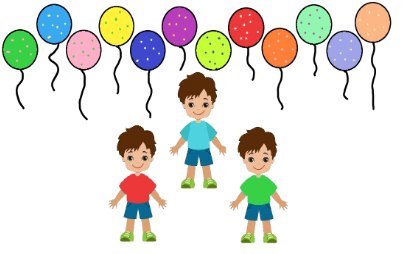_______ $$\div$$_______ $$=$$ _______ Each boy has _________ balloons.

Ans:

We need to share 12 balloons among 3 boys.

$$12 \div 3 = 4$$

Hence, each boy has $$4$$ balloons.

12. There are 21 candles. Put them equally in 3 boxes. How many candles are there in each box?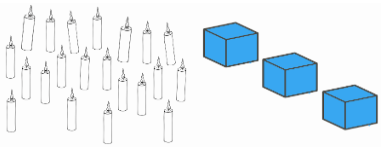Ans:

We need to put 21 candles are equally in 3 boxes.

Number of candles in each box $$21 \div 3 = 7$$

Hence, there are $$7$$ candles in each box.

13. There are 18 socks. How many girls can wear these socks?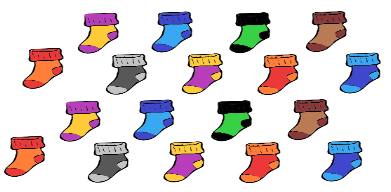Ans:

Number of socks available $$= 18$$

Number of socks can each girl wear $$= 2$$

So, number of girls who can wear 18 socks $$= 18 \div 2 = 9$$

Hence, $$9$$ girls can wear 18 shocks.

14. Raj has 36 minutes to make rotis. One roti takes 3 minutes. How many rotis can he make in this time? He can make ____________ rotis.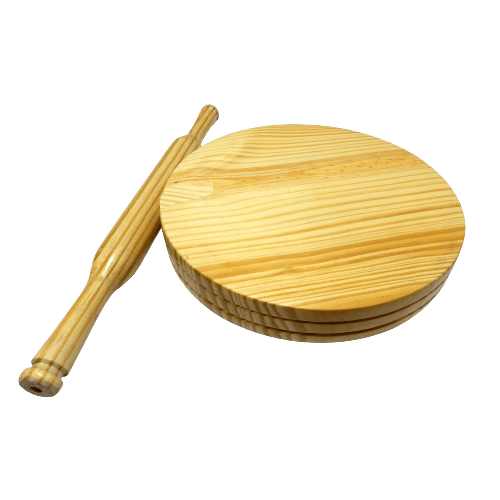Ans:

Raj has time to make rotis $$= 36$$ mins

Raj needs to prepare 1 roti $$= 3$$ mins

So, number of rotis can make in 36 mins $$= 36 \div 3 = 12$$

Hence, Raj can make $$12$$ rotis in $$36$$ minutes.

15. These are 24 footmarks of goats. So how many goats were there?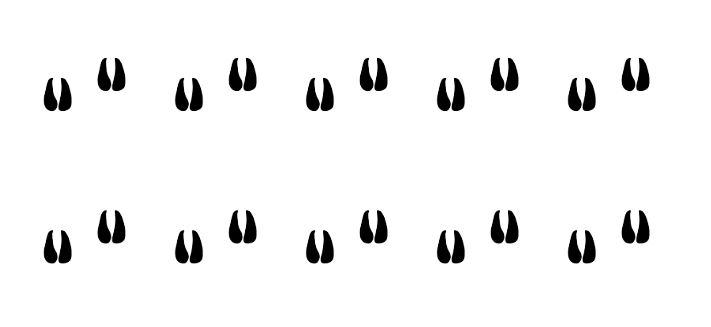Ans:

The number of foot a goat has $$= 4$$

Number of footmarks found in given figure $$= 24$$

Number of goats with 24 footmarks $$= 24 \div 4 = 6$$

Hence, there were $$6$$ goats.

16. Some girls are playing a game with both their hands. The girls who are playing have 60 fingers altogether. How many girls are playing this game?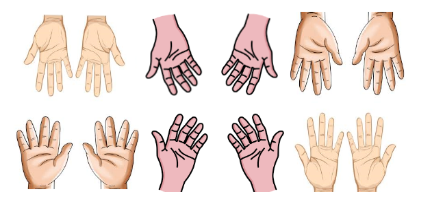Ans:

Number of fingers in the game (given figure) $$= 60$$

Number of fingers each girl has$$= 10$$

So, number girls having 60 fingers are$$= 60 \div 10 = 6$$

Hence, $$6$$ girls are playing a game with both their hands.

17. Lakshmi has 27 kg potatoes to sell. Three men came and bought equal amounts of potatoes. Each man bought __________ kg of potatoes.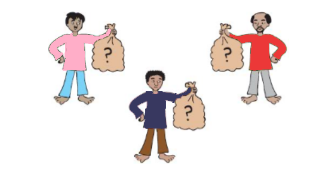Ans:

Number of potatoes Lakshmi has to sell $$= 27$$kgs

If 27 kg of potatoes are equally bought by three men then each man will get $$= 27 \div 3 = 9$$kg

Hence, each man has taken $$9$$ kg of potatoes.

18. Jumpy Animals.A frog jumps 2 steps at a time.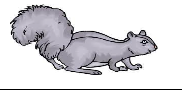A squirrel jumps 3 steps.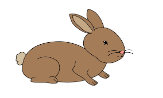A rabbit jumps 5 steps.A horse jumps 15 steps.A kangaroo jumps 30 steps.

19. Use the path on the next page to find out: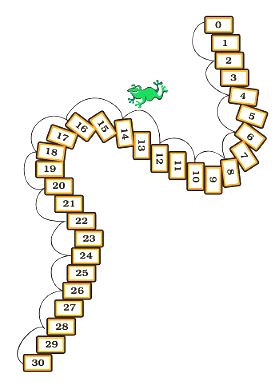1. In how many jumps will the frog reach 30? $$30 \div 2 =$$ _________

Ans: $$30 \div 2 = 15$$

Frog will take 15 jumps to reach 30 steps.

2. In how many jumps will the squirrel reach 27? $$27 \div 3 =$$ _________

Ans: $$27 \div 3 = 9$$

squirrel will take 9 jumps to reach 27 steps.

3. Which number will the kangaroo reach in two jumps?

Ans: we know that, Kangaroo takes 30 steps in one jump.

Thus, kangaroo will reach $$= 30 \times 2 = 60$$ steps in two jumps.

4. Who all will meet at the number 15? _________, _________, _________

Ans: The number 15 comes in the table of 3, 5, and 15.

Squirrel will reach 15 steps because$$= 3 \times 5 = 15$$.

Rabbit will reach 15 steps because $$= 5 \times 3 = 15$$.

Horse will reach 15 steps because $$= 15 \times 1 = 15$$.

Hence, squirrelrabbit, and horse will meet at number 15.

5. Will the rabbit ever be at the number 18? ________

Ans: Given, Rabbit's one jump is equal to 5 steps

Thus, Rabbit can never be at 18 steps because 18 does not comes in the table of 5.

6. How many jumps of the rabbit equal one jump of the horse? ________

Ans:

A horse takes 15 steps in one jump.

A rabbit takes 5 steps in one jump.

$$15 \div 3 = 15$$

Hence, 3 jumps of the rabbit equal to one jump of the horse.

7. How many jumps of the horse equals two jumps of the kangaroo?

Ans:

A horse takes 15 steps in one jump.

2 jumps of the kangaroo $$= 2 \times 30 = 60$$ steps.

$$60 \div 15 = 4$$

Hence, 4 jumps of the horse equals two jumps of the kangaroo.

8. Which is the smallest number where the frog and the squirrel will meet?

Ans:

Frog’s takes two steps in one jump. So, frog takes steps 2, 4, 6, 8 and so on.

Squirrel’s takes three steps in one jump. So, frog takes steps 3, 6, 9, 12 and so on.

Number 6 is the common step of both frog and squirrel.

Thus, the smallest number where frog and squirrel will meet is 6th step.

20. Divide into groups of 2 using a 2 times table.

 $$18$$ $$\div$$ $$2$$ $$=$$ $$9$$ Hint: $$2 \times 9 = 18$$ $$18$$ $$\div$$ $$=$$ $$2$$ $$16$$ $$\div$$ $$2$$ $$=$$ $$20$$ $$\div$$ $$2$$ $$=$$ $$\div$$ $$2$$ $$=$$ $$7$$ $$\div$$ $$2$$ $$=$$ $$10$$ $$8$$ $$\div$$ $$=$$ $$4$$ $$\div$$ $$2$$ $$=$$ $$5$$
Ans:
 $$18$$ $$\div$$ $$2$$ $$=$$ $$9$$ Hint: $$2 \times 9 = 18$$ $$18$$ $$\div$$ $$9$$ $$=$$ $$2$$ Hint: $$9 \times 2 = 18$$ $$16$$ $$\div$$ $$2$$ $$=$$ $$8$$ Hint: $$2 \times 8 = 16$$ $$20$$ $$\div$$ $$2$$ $$=$$ $$10$$ Hint: $$2 \times 10 = 20$$ $$14$$ $$\div$$ $$2$$ $$=$$ $$7$$ Hint: $$2 \times 7 = 14$$ $$20$$ $$\div$$ $$2$$ $$=$$ $$10$$ Hint: $$2 \times 10 = 20$$ $$8$$ $$\div$$ $$2$$ $$=$$ $$4$$ Hint: $$2 \times 4 = 8$$ $$10$$ $$\div$$ $$2$$ $$=$$ $$5$$ Hint: $$2 \times 5 = 10$$

21. Divide into groups of 5 using 5 times table.

 $$10$$ $$\div$$ $$5$$ $$=$$ Hint: $$5 \times 2 = ?$$ $$20$$ $$\div$$ $$=$$ $$4$$ $$15$$ $$\div$$ $$5$$ $$=$$ $$40$$ $$\div$$ $$=$$ $$8$$ $$20$$ $$\div$$ $$5$$ $$=$$ $$\div$$ $$5$$ $$=$$ $$6$$ $$25$$ $$\div$$ $$5$$ $$=$$ $$\div$$ $$5$$ $$=$$ $$3$$ $$35$$ $$\div$$ $$5$$ $$=$$ $$\div$$ $$5$$ $$=$$ $$2$$

Ans:

 $$10$$ $$\div$$ $$5$$ $$=$$ $$2$$ Hint: $$5 \times 2 = 10$$ $$20$$ $$\div$$ $$5$$ $$=$$ $$4$$ Hint: $$5 \times 4 = 20$$ $$15$$ $$\div$$ $$5$$ $$=$$ $$3$$ Hint: $$5 \times 3 = 15$$ $$40$$ $$\div$$ $$5$$ $$=$$ $$8$$ Hint: $$5 \times 8 = 40$$ $$20$$ $$\div$$ $$5$$ $$=$$ $$4$$ Hint: $$5 \times 4 = 20$$ $$30$$ $$\div$$ $$5$$ $$=$$ $$6$$ Hint: $$5 \times 6 = 30$$ $$25$$ $$\div$$ $$5$$ $$=$$ $$5$$ Hint: $$5 \times 5 = 25$$ $$15$$ $$\div$$ $$5$$ $$=$$ $$3$$ Hint: $$5 \times 3 = 15$$ $$35$$ $$\div$$ $$5$$ $$=$$ $$7$$ Hint: $$5 \times 7 = 35$$ $$10$$ $$\div$$ $$5$$ $$=$$ $$2$$ Hint: $$5 \times 2 = 10$$

22. Try these.

 $$4$$ $$\div$$ $$=$$ $$2$$ $$14$$ $$\div$$ $$7$$ $$=$$ $$6$$ $$\div$$ $$3$$ $$=$$ $$\div$$ $$2$$ $$=$$ $$7$$ $$\div$$ $$2$$ $$=$$ $$3$$ $$15$$ $$\div$$ $$3$$ $$=$$ $$8$$ $$\div$$ $$4$$ $$=$$ $$15$$ $$\div$$ $$5$$ $$=$$ $$8$$ $$\div$$ $$=$$ $$4$$ $$\div$$ $$2$$ $$=$$ $$8$$

 $$9$$ $$\div$$ $$3$$ $$=$$ $$18$$ $$\div$$ $$9$$ $$=$$ $$\div$$ $$2$$ $$=$$ $$5$$ $$20$$ $$\div$$ $$5$$ $$=$$ $$12$$ $$\div$$ $$4$$ $$=$$ $$20$$ $$\div$$ $$4$$ $$=$$ $$12$$ $$\div$$ $$=$$ $$2$$

Ans:

 $$4$$ $$\div$$ $$2$$ $$=$$ $$2$$ Hint: $$2 \times 2 = 4$$ $$14$$ $$\div$$ $$7$$ $$=$$ $$2$$ Hint: $$7 \times 2 = 14$$ $$6$$ $$\div$$ $$3$$ $$=$$ $$2$$ Hint: $$3 \times 2 = 6$$ $$14$$ $$\div$$ $$2$$ $$=$$ $$7$$ Hint: $$2 \times 7 = 14$$ $$6$$ $$\div$$ $$2$$ $$=$$ $$3$$ Hint: $$2 \times 3 = 6$$ $$15$$ $$\div$$ $$3$$ $$=$$ $$5$$ Hint: $$3 \times 5 = 15$$ $$8$$ $$\div$$ $$4$$ $$=$$ $$2$$ Hint: $$4 \times 2 = 8$$ $$15$$ $$\div$$ $$5$$ $$=$$ $$3$$ Hint: $$5 \times 3 = 15$$ $$8$$ $$\div$$ $$2$$ $$=$$ $$4$$ Hint: $$2 \times 4 = 8$$ $$16$$ $$\div$$ $$2$$ $$=$$ $$8$$ Hint: $$2 \times 8 = 16$$

 $$9$$ $$\div$$ $$3$$ $$=$$ $$3$$ Hint: $$3 \times 3 = 9$$ $$18$$ $$\div$$ $$9$$ $$=$$ $$2$$ Hint: $$9 \times 2 = 18$$ $$10$$ $$\div$$ $$2$$ $$=$$ $$5$$ Hint: $$2 \times 5 = 10$$ $$20$$ $$\div$$ $$5$$ $$=$$ $$4$$ Hint: $$5 \times 4 = 20$$ $$12$$ $$\div$$ $$4$$ $$=$$ $$3$$ Hint: $$4 \times 3 = 12$$ $$20$$ $$\div$$ $$4$$ $$=$$ $$5$$ Hint: $$4 \times 5 = 20$$ $$12$$ $$\div$$ $$6$$ $$=$$ $$2$$ Hint: $$6 \times 2 = 12$$

### NCERT Solutions for Class 3 Maths Chapter 12 Can We Share

As the name of Chapter 12– Can We Share suggests, this chapter is linked to division and distribution. This is a new concept. You can solve the sums given in the exercise and verify your answers with the NCERT Solutions for Class 3 Chapter 12 – Can We Share? to identify and correct your mistakes. You can go through these NCERT Solutions and prepare for your exams. You can also take the help of our experienced and friendly teachers if you have any doubts related to the topic.

NCERT Solutions for Class 3 Maths Chapter 12 – Can We Share? introduces the concept of division and distribution.

If we want to share something then we need to make equal parts. For example, your mother buys chocolate for you and she asks you to share with your sister. There are 8 pieces in the chocolate bar. How many does each get?

You and your sister get 4 pieces each. So, what are you doing here? You are making equal parts of the chocolate. This means splitting it into equal parts or groups or dividing it into equal parts.

Division means splitting or sharing into equal parts. The symbol of division is ‘ ÷ ‘.

Let us see an example.

In the above picture, there are 24 apples that need to be put equally in 6 boxes. How many apples will go into each box?

We have to distribute the apples in equal parts. So we write,

24 ÷ 6 = 4

The answer is 4 apples will go into each box. How did we get this answer? We have all 24 apples and we have divided the apples by the number of boxes.

The number to be divided is called the dividend. The number by which we divide the dividend is called the divisor. The result we get is called the quotient.

In the above example, 24 apples make the dividend. 6 boxes make the divisor, and the result, 4 apples is the quotient.

### Why Do We Divide by Six Boxes?

We know the multiplication: 6 x 4 = 24. Here, 6 and 4 are called the factors of 24. 24 is called the multiple.

When we divide the multiple by one of the factors we get the other factor as the result. Hence you can say that division is opposite of multiplication.

Do we have to know tables to do division? Yes, you have to know the tables to do division. It will make our calculation faster.

### Other Ways of Distribution

We can also distribute these 24 apples in other ways. We can divide the apples into 8 equal parts or 3 equal parts or 2 equal parts or 12 equal parts.

Let us see how many boxes we need in each kind of distribution.

• If we divide the apples into 8 equal parts then we need 3 boxes.

• If we divide the apples into 3 equal parts then we need 8 boxes.

• If we divide the apples into 12 equal parts then we need 2 boxes.

• If we divide the apples into 2 equal parts then we need _______ boxes? Can you guess?

### Conclusion

With the help of this concept, now students can solve all the exercise questions given in the chapter and also verify them with the NCERT Solutions Maths Chapter 12. ‘Division’ is one of the fundamental mathematical operations. It is one of the basic skills that you need in your daily life. With the help of the concept of division, you can distribute time for all your daily activities, wisely. This concept will help when you will learn to count money as well.

## FAQs on NCERT Solutions for Class 3 Maths Chapter 12

1. What do you understand by the term ‘division’?

Division means splitting or distributing anything into equal parts.

2. Why should I choose Vedantu to prepare for the exams?

The concepts for NCERT Solutions for Maths for Class 3 are very well developed and very comprehensive in the NCERT Solutions available on Vedantu, to help students understand the concept in a very simple way. Our study materials give a very clear idea about the concepts and help students to develop a strong conceptual foundation. The Class 3 NCERT Solutions cover all the exercises from the NCERT books in Maths.

3. How do you avail of the NCERT Solutions for Class 3 Maths?

NCERT Solutions for Class 3 Maths and its reference notes per topic are available on Vedantu. They are available in PDF format and you can download them on your smartphones, computers, laptop, and any other device. Our subject-matter experts have researched extensively and developed the NCERT Solutions Maths in a step-by-step method making it self-explanatory for all students. The students can revise and solve as many questions as possible to master this topic.

4. Why Vedantu is trusted by so many students?

Vedantu is an excellent academic platform where you can find skilled and experienced teachers and subject experts. Students can download NCERT Solutions Class 3 Maths PDF for free from Vedantu. Students can easily access these materials to develop their understanding of the concepts of various topics. Our teachers are always there to address the doubts of the students. They are very friendly and they are experienced to understand the pulse of the students and guide them accordingly. Vedantu is a very user-friendly platform and students can avail of the benefits from anywhere in the world at their convenient time.

5. What does Chapter 12 “Can We Share” of Class 3 Maths teach us?

Chapter 12 “Can We Share” of Class 3 Maths enables the students to understand the concept of distribution and division. This chapter explains all the necessary information in simple language with daily life examples so that the kids can sketch an outline of the chapter. Additionally, the chapter also includes interesting activities that excite the student to read the given content.

6. Where can you get the NCERT Solutions for Chapter 12 “Can We Share” of Class 3 Maths online?

You can get the NCERT Solutions for Chapter 12 “Can We Share” of Class 3 Maths free of cost on the Vedantu website and the Vedantu app. Here, you will find notes and a summary of the chapter in easy language, along with textbook questions and answers. At the same time, you can also discover some important terms of the chapter written interestingly so that the kids can relate themselves to those terms.

7. What are the benefits of reading NCERT Solutions for Chapter 12 of Class 3 Maths?

As a Class 3 student, reading NCERT Solutions of Chapter 12 “Can We Share” can help you in different ways like:

• These solutions will assist you in understanding the topic clearly.

• They give a better outlook of the chapter.

• They help to comprehend the crucial terms and concepts efficiently.

• The NCERT Solutions aid you to score good marks in your tests.

8. Is Chapter 12 of Class 3 Maths easy?

For some students, the chapter is easy. However, some students may face a few problems. To avoid this issue, a student must attend all the classes for different subjects regularly. They should concentrate on their lectures when the teacher is teaching in the class and revise the chapter at home. They should ask their queries from their mentors to clear the concepts.

9. How to teach division to Class 3 students?

If you want to teach division to Class 3 students, then follow the listed tips.

• First, try to teach division, including the even numbers. Dividing even numbers is easier as compared to dividing odd numbers.

• Try to explain the important terms of the chapter, including dividends, remainders, divisors, with the help of images, videos, or some interesting daily based activities.SHARETWEETSHARESUBSCRIBE